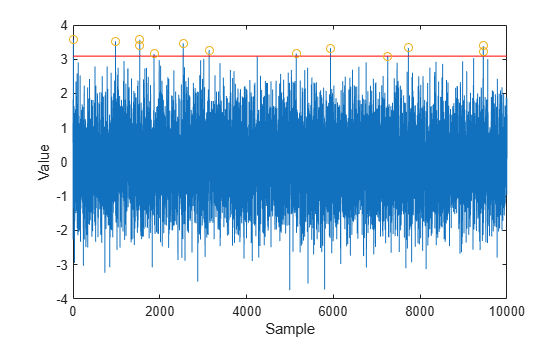## Neyman-Pearson Hypothesis Testing

### Purpose of Hypothesis Testing

In phased-array applications, you sometimes need to decide between two competing hypotheses to determine the reality underlying the data the array receives. For example, suppose one hypothesis, called the null hypothesis, states that the observed data consists of noise only. Suppose another hypothesis, called the alternative hypothesis, states that the observed data consists of a deterministic signal plus noise. To decide, you must formulate a decision rule that uses specified criteria to choose between the two hypotheses.

### Support for Neyman-Pearson Hypothesis Testing

When you use Phased Array System Toolbox™ software for applications such as radar and sonar, you typically use the Neyman-Pearson (NP) optimality criterion to formulate your hypothesis test.

When you choose the NP criterion, you can use `npwgnthresh` to determine the threshold for the detection of deterministic signals in white Gaussian noise. The optimal decision rule derives from a likelihood ratio test (LRT). An LRT chooses between the null and alternative hypotheses based on a ratio of conditional probabilities.

`npwgnthresh` enables you to specify the maximum false-alarm probability as a constraint. A false alarm means determining that the data consists of a signal plus noise, when only noise is present.

For details about the statistical assumptions the `npwgnthresh` function makes, see the reference page for that function.

### Threshold for Real-Valued Signal in White Gaussian Noise

This example shows how to compute empirically the probability of false alarm for a real-valued signal in white Gaussian noise.

Determine the required signal-to-noise (SNR) in decibels for the NP detector when the maximum tolerable false-alarm probability is 10^-3.

```Pfa = 1e-3; T = npwgnthresh(Pfa,1,'real');```

Determine the actual detection threshold corresponding to the desired false-alarm probability, assuming the variance is 1.

```variance = 1; threshold = sqrt(variance * db2pow(T));```

Verify empirically that the detection threshold results in the desired false-alarm probability under the null hypothesis. To do so, generate 1 million samples of a Gaussian random variable, and determine the proportion of samples that exceed the threshold.

```rng default N = 1e6; x = sqrt(variance) * randn(N,1); falsealarmrate = sum(x > threshold)/N```
```falsealarmrate = 9.9500e-04 ```

Plot the first 10,000 samples. The red horizontal line shows the detection threshold.

```x1 = x(1:1e4); plot(x1) line([1 length(x1)],[threshold threshold],'Color','red') xlabel('Sample') ylabel('Value')```You can see that few sample values exceed the threshold. This result is expected because of the small false-alarm probability.

### Threshold for Two Pulses of Real-Valued Signal in White Gaussian Noise

This example shows how to empirically verify the probability of false alarm in a system that integrates two real-valued pulses. In this scenario, each integrated sample is the sum of two samples, one from each pulse.

Determine the required SNR for the NP detector when the maximum tolerable false-alarm probability is $1{0}^{-3}$.

```pfa = 1e-3; T = npwgnthresh(pfa,2,'real');```

Generate two sets of one million samples of a Gaussian random variable.

```rng default variance = 1; N = 1e6; pulse1 = sqrt(variance)*randn(N,1); pulse2 = sqrt(variance)*randn(N,1); intpuls = pulse1 + pulse2;```

Compute the proportion of samples that exceed the threshold.

```threshold = sqrt(variance*db2pow(T)); falsealarmrate = sum(intpuls > threshold)/N```
```falsealarmrate = 9.8900e-04 ```

The empirical false alarm rate is very close to .001

### Threshold for Complex-Valued Signals in Complex White Gaussian Noise

This example shows how to empirically verify the probability of false alarm in a system that uses coherent detection of complex-valued signals. Coherent detection means that the system utilizes information about the phase of the complex-valued signals.

Determine the required SNR for the NP detector in a coherent detection scheme with one sample. Use a maximum tolerable false-alarm probability of $1{0}^{-3}$.

```pfa = 1e-3; T = npwgnthresh(pfa,1,'coherent');```

Test that this threshold empirically results in the correct false-alarm rate The sufficient statistic in the complex-valued case is the real part of the received sample.

```rng default variance = 1; N = 1e6; x = sqrt(variance/2)*(randn(N,1)+1j*randn(N,1)); threshold = sqrt(variance*db2pow(T)); falsealarmrate = sum(real(x)>threshold)/length(x)```
```falsealarmrate = 9.9500e-04 ```